# 深度学习火了那么多年，到底怎么搞？使用Numpy快速入门

1.  深度学习基础知识
2.  Numpy实现神经网络构建和梯度下降算法
3.  计算机视觉领域主要方向的原理、实践
4.  自然语言处理领域主要方向的原理、实践
5.  个性化推荐算法的原理、实践

1.  人工智能、机器学习、深度学习三者的关系，并简要介绍了深度学习的发展历史以及未来趋势。
2.  介绍构建深度模型的五个步骤，并使用Numpy实现神经网络。
3.  原理介绍和代码实践并行，详细介绍了使用Numpy实现梯度下降算法。

1.  人工智能、机器学习、深度学习三者之间关系是什么？
2.  一般的机器学习方法是什么？
3.  为什么那么多人看好深度学习，其未来的发展趋势是什么？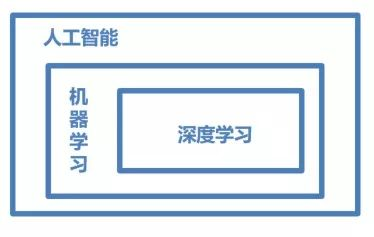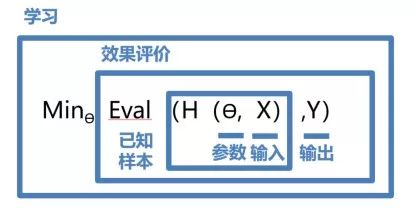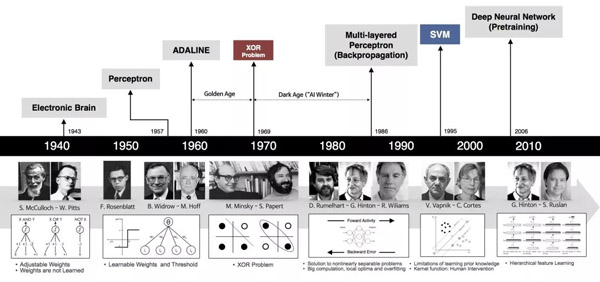•  数据处理：从本地文件或网络地址读取数据，并做预处理操作，如校验数据的正确性等。
•  模型设计：完成网络结构的设计（模型要素1），相当于模型的假设空间，即模型能够表达的关系集合。
•  训练配置：设定模型采用的寻解算法（模型要素2），即优化器，并指定计算资源。
•  训练过程：循环调用训练过程，每轮均包括前向计算 、损失函数（优化目标，模型要素3）和后向传播这三个步骤。
•  保存模型：将训练好的模型保存，以备预测时调用。

2. # 从文件导入数据
3.     datafile = './work/housing.data'
4.     data = np.fromfile(datafile, sep=' ')
5. # 每条数据包括14项，其中前面13项是影响因素，第14项是相应的房屋价格中位数
6.     feature_names = [ 'CRIM', 'ZN', 'INDUS', 'CHAS', 'NOX', 'RM', 'AGE', \
7. 'DIS', 'RAD', 'TAX', 'PTRATIO', 'B', 'LSTAT', 'MEDV' ]
8.     feature_num = len(feature_names)
9. # 将原始数据进行Reshape，变成[N, 14]这样的形状
10.     datadata = data.reshape([data.shape // feature_num, feature_num])
11. # 将原数据集拆分成训练集和测试集
12. # 这里使用80%的数据做训练，20%的数据做测试
13. # 测试集和训练集必须是没有交集的
14.     ratio = 0.8
15.     offset = int(data.shape * ratio)
16.     training_data = data[:offset]
17. # 计算train数据集的最大值，最小值，平均值
18.     maximums, minimums, avgs = training_data.max(axis=0), training_data.min(axis=0), \
19.                                  training_data.sum(axis=0) / training_data.shape
20. # 对数据进行归一化处理
21. for i in range(feature_num):
22. #print(maximums[i], minimums[i], avgs[i])
23.         data[:, i] = (data[:, i] - avgs[i]) / (maximums[i] - minimums[i])
24. # 训练集和测试集的划分比例
25.     training_data = data[:offset]
26.     test_data = data[offset:]
27. return training_data, test_data

1. class Network(object):
2.     def __init__(self, num_of_weights):
3.         # 随机产生w的初始值
4.         # 为了保持程序每次运行结果的一致性，
5.         # 此处设置固定的随机数种子
6.         np.random.seed(0)
7.         self.w = np.random.randn(num_of_weights, 1)
8.         self.b = 0.
9.     def forward(self, x):
10.         z = np.dot(x, self.w) + self.b

Numpy实现梯度下降算法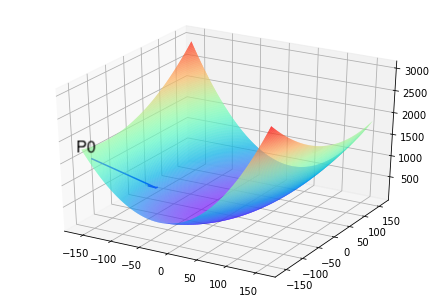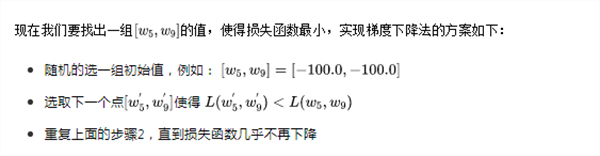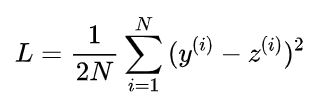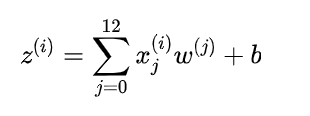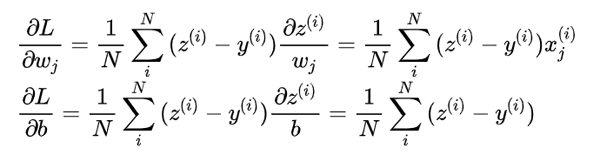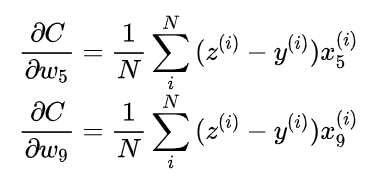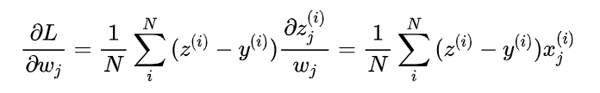2.     z = self.forward(x)
6.     gradient_b = (z - y)
9. def update(self, graident_w5, gradient_w9, eta=0.01):
10.     net.w = net.w - eta * gradient_w5
11.     net.w = net.w - eta * gradient_w9
12. def train(self, x, y, iterations=100, eta=0.01):
13.     points = []
14.     losses = []
15.     for i in range(iterations):
16.         points.append([net.w, net.w])
17.         z = self.forward(x)
18.         L = self.loss(z, y)
23.         losses.append(L)
24.         if i % 50 == 0:
25.             print('iter {}, point {}, loss {}'.format(i, [net.w, net.w], L))
26.     return points, losses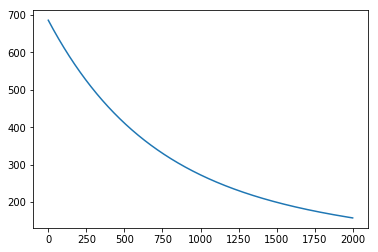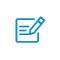扫码入群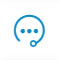咨询反馈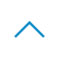返回顶部